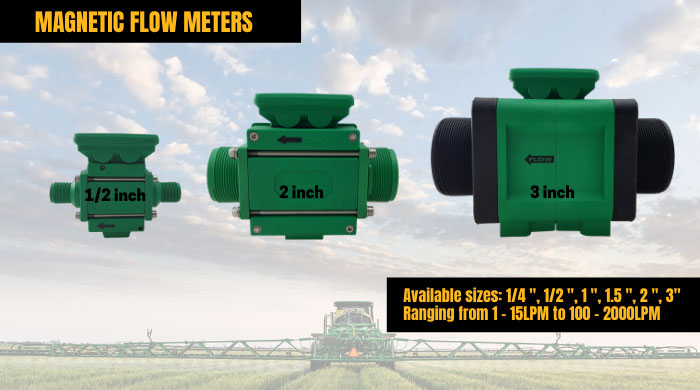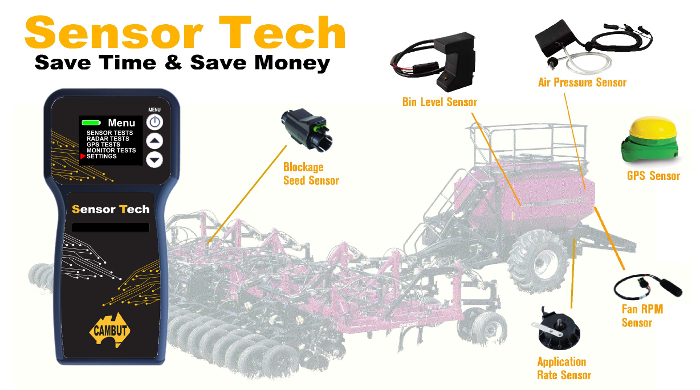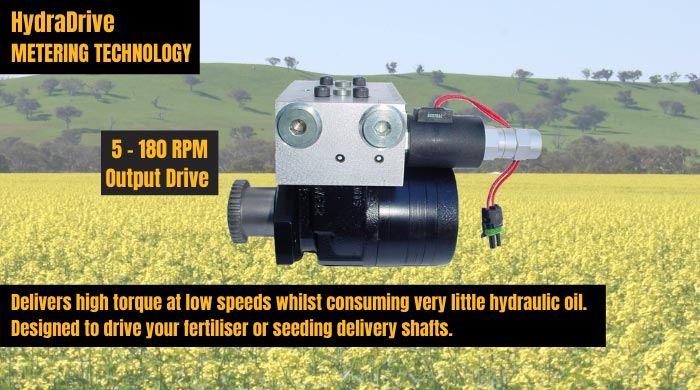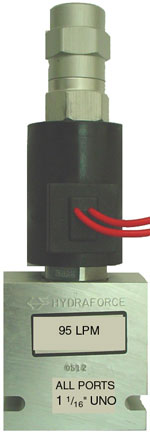# Hydraulic Valves/Motors## Hydraforce PWM Valves

Description :

LIQUID FLOW RATE FORMULA

To calculate liquid flow rates (gpm) so you can pick the correct Flow Control Valve for your application, use the following formula:

Width x speed x application rate (gpa) x 0.00202 = gpm

Example: 60 ft. x 15 mph x 20 gpa x 0.00202 = 36.36 gpm